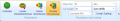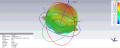# calculating vissually axial ratio frm radiation pattern

#### yef smith

Joined Aug 2, 2020
602
Hello,In anechoic chamber we are testing the antenna using a test antenna,in our case if we design circular patch antenna then we test it with circular antenna.In cst i think this option is as shown bellow.It gives me some radiation pattern as shown bellow.
I dont want to use the axial ratio option in CST,I want to calculate the axial ratio from the radiation pattern.
Axial ratio by definition is the ratio between electric field components.
How do i see the axial ratio from the radiation pattern shown bellow?
Thanks.#### Tesla23

Joined May 10, 2009
537
In that
I dont want to use the axial ratio option in CST,I want to calculate the axial ratio from the radiation pattern.
In that case you need to work it out from the relative amplitudes and the relative phases of the two orthogonal linear polarizations. Check out the definition of circular polarization.